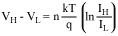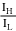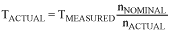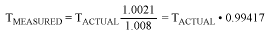# Compensating for Ideality Factor and Series Resistance Differences between Thermal-Sense Diodes

### Abstract

When using an external thermal diode to measure temperature, the accuracy of the temperature measurement depends on the characteristics of the external diode. Two critical parameters that affect measurement accuracy are ideality factor and series resistance. This application note explains the effects of these parameters on remote temperature-sensor measurements and discusses how to determine compensation factors for their effects.

The most common approach to measuring temperature with a "remote-diode" temperature sensor is to force two different currents through the diode¹, typically with a current ratio of about 10:1. The diode's voltage is measured at each current level and the temperature is calculated based on the equation:Where:
IH is the larger diode bias current.
IL is the smaller diode bias current.
VH is the diode voltage while IH is flowing.
VL is the diode voltage while IL is flowing.
n is the ideality factor of the diode (nominally 1, but varies with processing).
k is Boltzmann's constant (1.38 × 10-23joules/K).
T is the temperature in K.
q is the charge of an electron (1.60 × 10-19C)

If= 10, this can be simplified to:

VH - VL = 1.986 × 10-4 × πT

### Ideality Factor Correction

Note that the accuracy of the temperature reading depends on the value of n. If the remote diode sensor is designed to produce correct readings with a diode that has a specific value of n, then changing to a diode with a different ideality factor will change the apparent measured temperature.

Correcting for differences in ideality factor is done as follows. Assume that a remote diode sensor designed for a nominal ideality factor, nNOMINAL, is used to measure the temperature of a diode with a different ideality factor, nACTUAL. The measured temperature, TMEASURED, can be corrected using:Where T is the temperature in K.

Most remote diode temperature sensors for CPUs are designed to produce accurate temperature data when used with an ideality factor of 1.008. Some newer CPU thermal-sense diodes have lower ideality factors. To use a CPU optimized for an ideality factor of 1.008 with a CPU that has an ideality factor of 1.0021, the data can be corrected (assuming no series resistance) as follows:For an actual temperature of 85°C (358.15K), the measured temperature will be 82.91°C (356.06K), an error of -2.09°. Note that the error is proportional to absolute temperature. At 125°C, the error increases to -2.32°.

### Series Resistance Correction

Series resistance in one of the diodes contributes additional errors. For the nominal diode currents of 10µA and 100µA used in Maxim's remote temperature sensors, the change in the measured voltage will be:

RS(100µA - 10µA) = 90µA × RS

Since 1°C corresponds to 198.6µV, series resistance contributes a temperature offset of:Assume that the diode being measured has a series resistance of 3.86Ω. The series resistance contributes an offset of:

3.86Ω × 0.453°C/Ω = 1.75°C

If the diode has an ideality factor of 1.0021 and series resistance of 3.86Ω, the total offset can be calculated as follows. Combining the correction for series resistance with the correction for ideality factor, we have:

1.75°C - 2.09°C = -0.34°C

This is for a diode temperature of 85°C. Thus, in this case the effect of the series resistance and the ideality factor partially cancel each other.

Note that if the diode bias current is different, the effect of series resistance will change proportionally. For example, some remote temperature sensors have diode bias currents two or more times larger than those of Maxim's remote sensors. The resulting temperature errors can be on the order of two or more degrees larger than those observed with Maxim's sensors.

Some temperature sensors include automatic series resistance cancellation within their remote-diode sensing circuitry. When this function is enabled, these sensors bias the external diodes with three or four different current levels and use the resulting voltage measurements to eliminate the effect of series resistance from the temperature calculation. The MAX6654 and MAX6690 temperature sensors have a single remote channel with optional series resistance cancellation. Several multichannel remote sensors, including the MAX6602, MAX6689, MAX6697, MAX6698, and MAX6699, have series resistance cancellation on one of the remote channels. The MAX6581, with seven remote channels, includes series resistance cancellation on all remote channels.

¹This diode is not a two-lead rectifier or signal diode like a 1N4001. Such diodes will not work with remote-diode temperature sensors. Instead, the diode is really a bipolar transistor connected as a diode. If the transistor is a discrete unit, its base and collector should be connected together. If the transistor is a substrate PNP, the collector will be grounded and the base and emitter serve as the cathode and anode. When "diode" is used in this document, it refers to the diode-connected transistors described above.